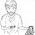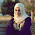Hello World!

Hello World!

Hello everyone, I'm creating this blog with the purpose of sharing my and your ideas with all hungry for game development.

Sunday, February 20, 2011

Roll a Cube.

I'm working in a game type which  the main scope is to roll a cube, i was searching methods to do this, and the best way which  i could  found is the method described by HiggyB in unity answers forum ( http://forum.unity3d.com/threads/16190-How-to-move-camera-automatically/page2). So let's Work!

Download de Project here, our create a cube and a javascript ,copy and paste the follow code and attach to the cube.

private var ismoving : boolean = false;
private var startY : float = 0;
var cubeSpeed : float;
var cubeSize: int;

function Update ()
{

if (Input.GetKeyDown("up") && ismoving == false)
{
ismoving = true;
transform.Find("targetpoint").Translate(0, -cubeSize/2 , cubeSize/2);
StartCoroutine(DoRoll(transform.Find("targetpoint").position, Vector3.right, 90.0f,cubeSpeed));
}
if (Input.GetKeyDown("down") && ismoving == false)
{
ismoving = true;
transform.Find("targetpoint").Translate(0, -cubeSize/2, -cubeSize/2);
StartCoroutine(DoRoll(transform.Find("targetpoint").position, -Vector3.right, 90.0f,cubeSpeed));
}
if (Input.GetKeyDown("left") && ismoving == false)
{
ismoving = true;
transform.Find("targetpoint").Translate(-cubeSize/2, -cubeSize/2, 0);
StartCoroutine(DoRoll(transform.Find("targetpoint").position, Vector3.forward, 90.0,cubeSpeed));
}
if (Input.GetKeyDown("right") && ismoving == false)
{
ismoving = true;
transform.Find("targetpoint").Translate(cubeSize/2, -cubeSize/2, 0);
StartCoroutine(DoRoll(transform.Find("targetpoint").position, -Vector3.forward, 90.0f,cubeSpeed));
}
}

function DoRoll (aPoint, aAxis, aAngle, aDuration) {

var tSteps = Mathf.Ceil(aDuration * 30.0);
var tAngle = aAngle / tSteps;
var pos : Vector3; // declare variable to fix the y position

// Rotate the cube by the point, axis and angle
for (var i = 1; i <= tSteps; i++)
{
transform.RotateAround (aPoint, aAxis, tAngle);
yield WaitForSeconds(0.033333);
}

// move the targetpoint to the center of the cube
transform.Find("targetpoint").position = transform.position;

// Make sure the y position is correct
pos = transform.position;
pos.y = startY;
transform.position = pos;

// Make sure the angles are snaping to 90 degrees.
var vec = transform.eulerAngles;
vec.x = Mathf.Round(vec.x / 90) * 90;
vec.y = Mathf.Round(vec.y / 90) * 90;
vec.z = Mathf.Round(vec.z / 90) * 90;
transform.eulerAngles = vec;

// The cube is stoped
ismoving = false;
}

Select the cube and go to Menu GameObjec > Create Empty, rename it with the name targetpoint. In the Hierarchy tab, click and drag the new empty Object to the cube to make him your parent. Create a Javascript with the follow code and drag to targepoint object.

var gizmocolor : Color;
var Radius : float;

function Update () {
transform.rotation = Quaternion.identity;
}

function OnDrawGizmos(){
Gizmos.color = gizmocolor;
}

Dont forget to give values to the variables in the editor.

In the next post I intend to show how to load parameters from a xml file.
I hope you enjoy it, give your comments!

1.nice tutorial
thanks man

2.Hello, do you know if I can reuse this code but using forces instead of translations to move my cube and having axis constraints to stabilize the movement?

Thanks

3.Great help tutorial.
BUT: it not works on Android:
Assets/Scripts/RollCube.js(37,35): BCE0051: Operator '*' cannot be used with a left hand side of type 'Object' and a right hand side of type 'float'.

Code:
var tSteps = Mathf.Ceil(aDuration* 30.0);

1.casting should b done.
function DoRoll (aPoint, aAxis, aAngle: int, aDuration: float) {

var tSteps = Mathf.Ceil(aDuration * 30.0);
var tAngle = aAngle / tSteps;

4.if i change the dimension of the cube to (1*2*1) then the rotation changes a bit... can you check it

1.There is a solution here : https://pastebin.com/zFZEMF55

You have to create a quad for floor or mesh grill, and position the player ever your want;
Attach Rigidbody to take care of collision.

5.Hi, great tut,

How can I change the code to make the roll automatically, because when I leave the if Condition out, the cube spins super faster and makes a cercle formation

Thanks

1.Hello, Creating a bool variable called automatic = true; for example.

and then a coroutine instead of the function
ienumerator Roll(...)

in the end of coroutine:

if(automatic)
startcoroutine(Roll(...));

6.Hi,
Thank you very much for yout help it works now. I had actually made a Coroutine out of the function at first. But there is a point that I don’t understand. I removed the if statement to enable the Start of the couritine in the update function and this made the cube spinn completely wrong. Why does it work now, by putting an if statement which tells exactly the same thing (bool variable is always true)?

1.by starting the coroutine in the update function, you are calling the coroutine many times before this is complete finished. if you restart the coroutine at the end, you will execute once at time.

7.Can somebody please convert this script to c# ?

1.8.9.